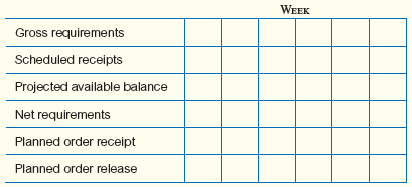# [Solved] Note: for These Problems, to Simplify Data Handling to Include

## Note: For these problems, to simplify data handling to include the receipt of orders that have actually been placed in previous periods, the following six-level scheme can be used. (A number of different techniques are used in practice, but the important issue is to keep track of what is on hand, what is expected to arrive, what is needed, and what size orders should be placed.) One way to calculate the numbers is as follows:Assume that Product Z is made of two units of A and four units of B. A is made of three units of C and four of D. D is made of two units of E.Lead times for purchase or fabrication of each unit to final assembly are: Z takes two weeks; A, B, C, and D take one week each; and E takes three weeks.Fifty units are required in Period 10. (Assume that there is currently no inventory on hand of any of these items.)a. Show the bill-of-materials (product structure tree).b. Develop an MRP planning schedule showing gross and net requirements and order release and order receipt dates.

Tags
Choose question tag10+ million students use Quizplus to study and prepare for their homework, quizzes and exams through 20m+ questions in 300k quizzes.

Explore our library and get Decision Science Homework Help with various study sets and a huge amount of quizzes and questions

### Related Questions

Get Free Access Now!